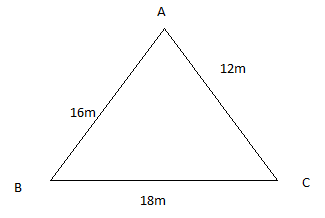QuestionAnswers

# Find the cost of fencing the ground in the form of a triangle with sides 16 m, 12 m and 18 m. The rate of fencing is Rs 25 per meter.

Hint: The dimensions of the triangular field are given. In order to find the length of the fence, one needs to find the perimeter of this field first. Now, the rate of fencing per meter length is given. Hence we can find the total cost of fencing the ground.The perimeter of the triangular field is $\left( {16 + 12 + 18} \right) = 46$ m.
Therefore in order to fence 46m, the total cost of fencing will be $46X25 = 1150$Rs.